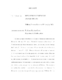## Riemannian Submersions And Lie Groups##### Files
umi-umd-2560.pdf(274.56 KB)
The principal topic of this thesis is the study of Riemannian submersions and Riemannian foliations of Lie groups. We classify Riemannian submersions of tori $T$ with left invariant (hence bi-invariant) metric. In this case, the fiber through the identity $F_e$ of the torus is a subgroup of $T$, and the submersion itself must be the coset projection $T \rightarrow T/F_e$. Then we characterize Riemannian submersions $f: G \rightarrow H$ where $G$ and $H$ are Lie groups with left invariant metric. These are homomorphisms if and only if the basic lift of a left invariant vector field in $H$ is a left invariant vector field in $G$. A similar theorem is proven to characterize antihomomorphisms. We then study a Ricatti equation associated with a Riemannian foliation, giving new proofs of several results of Walschap. We also give a relationship that must hold between the fiber dimension and base dimension of a Riemannian submersion, thus partially answering a question of Wilhelm. We then turn to the study of homogeneous Riemannian foliations. First we give a sufficient condition for a Riemannian foliation to be homogeneous, and use it to show that any Riemannian submersion of a compact Lie group with totally geodesic connected fibers is homogeneous if the fiber through the identity is a Lie subgroup. This is a result related to work of Ranjan \cite{Ranjan2}. Finally, we discuss the homogeneity of flat foliations of symmetric spaces of compact type, culminating in a proof that compact simple Lie groups with bi-invariant metric do not admit Riemannian foliations of codimension 1 with closed leaves.